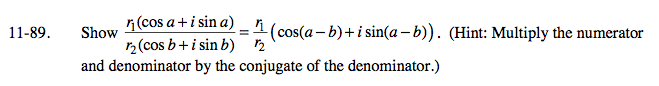### Home > PC > Chapter 11 > Lesson 11.2.2 > Problem11-89

11-89.$\frac{\textit{r}_1(\cos \textit{a}+\textit{i}\sin \textit{a})}{\textit{r}_2(\cos \textit{b}+\textit{i}\sin \textit{b})}·\frac{(\cos \textit{b}-\textit{i}\sin \textit{b})}{(\cos \textit{b}-\textit{i}\sin \textit{b})}$

$\frac{\textit{r}_1(\cos \textit{a} \cos \textit{b}-\textit{i}\cos \textit{a} \sin \textit{b} +\textit{i}\sin \textit{a} \cos \textit{b}-\textit{i}^2\sin \textit{a} \sin \textit{b})}{\textit{r}_2(\cos \textit{b} \cos \textit{b}-\textit{i}\cos \textit{b} \sin \textit{b} +\textit{i}\sin \textit{b} \cos \textit{b}-\textit{i}^2\sin \textit{b} \sin \textit{b})$

$\frac{\textit{r}_1\left( (\cos \textit{a} \cos \textit{b} +\sin \textit{a} \sin \textit{b})+\textit{i}(\sin \textit{a} \cos \textit{b}-\cos \textit{a} \sin \textit{b}) \right) }{\textit{r}_2(\cos^2 \textit{b}+\textit{i}sin^2 \textit{b})$

Use your trig identities to simpify.